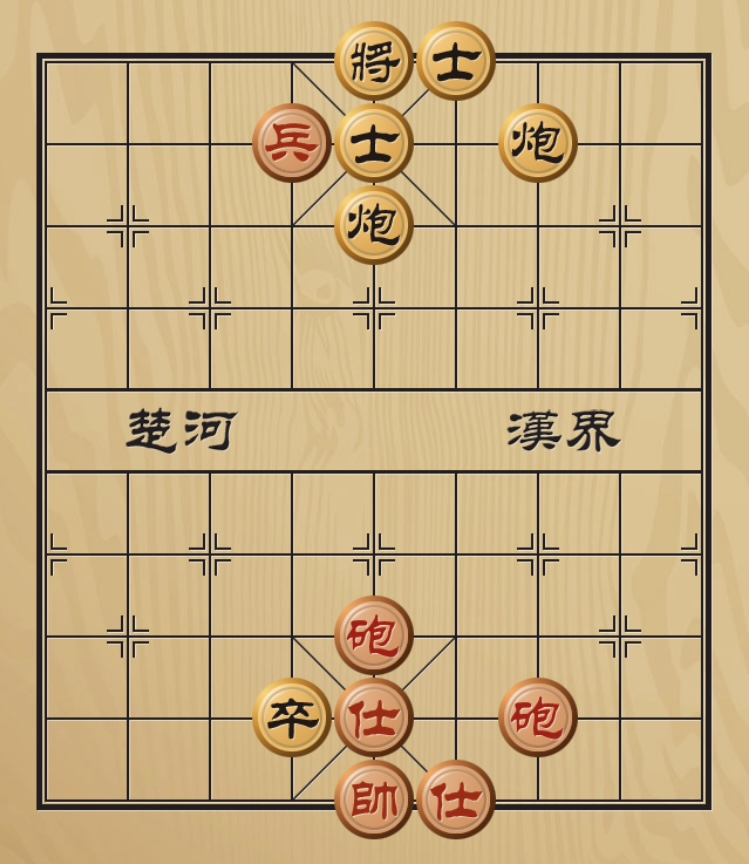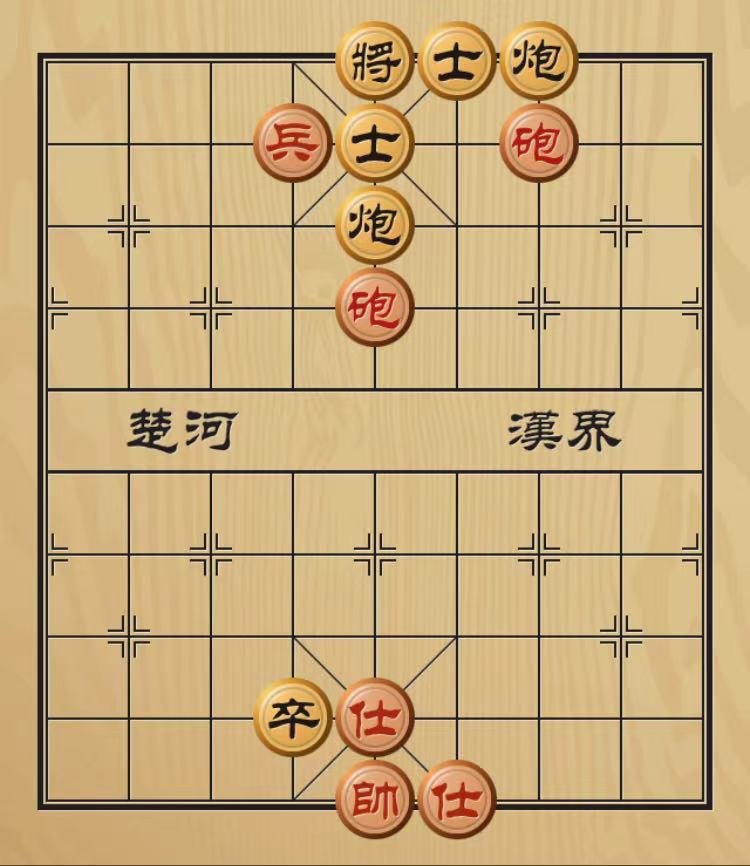# 一个象棋问题的扩展$$n=1$$时，起手红执第$$1$$路炮向前走$$x_1$$。

$$n=2$$时，起手红执第$$2$$路炮向前走$$(x_2 - x_1)$$步，此时$$x_2' = x_1$$。